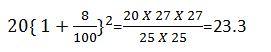# Compound Interest : 8 Questions that are Repeated in the Exams

Compound interest is an important for competitive examinations point of view. I am analyzing question papers of various examinations from past many years. I found that few questions are repeating in every exam. Today I am sharing 8 questions that are repeated in almost all the exams.

#### Ques 1.

If Inflation increases at a rate of 8 p.c.p.a What will a Rs.20 article cost at the end of two years ?
(a) between Rs,20 and Rs.21
(b) between Rs.21 and Rs.22
(c) between Rs.22 and Rs.23
(d) between Rs.23 and Rs.24
Ans 1..(d) Required sum =#### Ques 2.

What sum invested for 2 years at 12% compounded annually will glow to Rs.4390.40?
(a) Rs.4000
(b) Rs.3500
(c) Rs.3800
(d) Rs.3875
Ans 2.#### Ques 3.

What sum of money will become Rs.1352 in 2 years at 4 percent per annul compound interest ?
(a) Rs.1200
(b) Rs.1225
(c) Rs.1250
(d) Rs.1300
Ans 3.#### Ques 4.

What sum lent at 5%per annul compound interest will amount to Rs. 441 in 2 years?
(a) Rs. 390
(b) Rs. 395
(c) Rs.400
(d) Rs.405
Ans  4.#### Ques 5.

The principal that amount to Rs.4913 in 3 years at per annul compound interest compounded annually is
(a) Rs.4096
(b) Rs.4085
(c) Rs.4076
(d) Rs.3096
Ans 5.

#### Ques 6.

If the total amount interest earned after 2 years at the rate of 12%per annual is Rs.228.96, then the principal amount is
(a) 1,200
(b) 1,100
(c) 1,000
(d) 900
Ans 6.   Let P be the principal#### Ques 7.

If a sum of Rs.1000 deposited at compound interest double afteer 4 years .After 20 years, it will become
(a) Rs.20,000
(b) Rs 24,000
(c) Rs.28,000
(d) Rs.32,000
Ans 7. = ( d )

#### Ques 8.

If Raja paid total amount of Rs.324.48 after 2 years with compound interest at 4% per annul,then the principal amounts taken by Raja must be
(a) Rs.300
(b) Rs.320
(c) Rs.310
(d) Rs.316
Ans 8. = ( a )